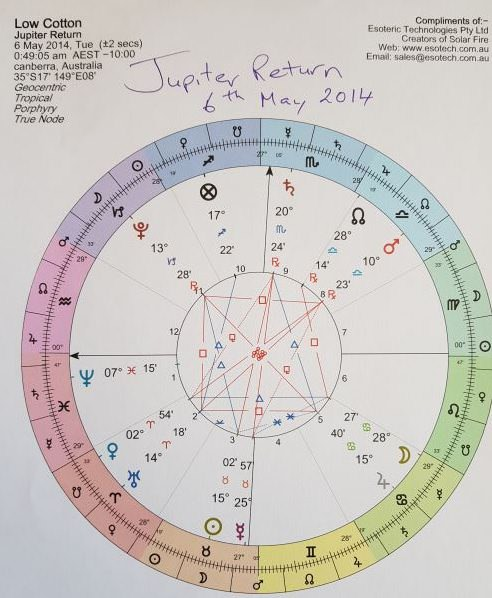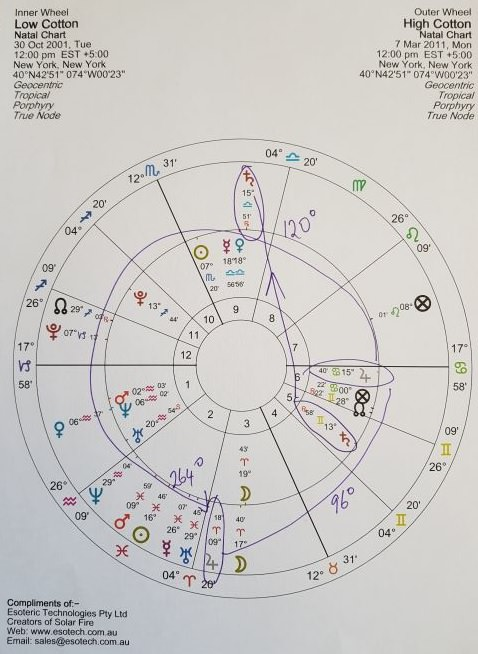W.D.Ganns Squaring Time And PriceMost have heard of W.D.Ganns squaring TIME and PRICE, but what does it mean?D.K.Burton

If we take a low, which is the first place to start and convert that price to time we are really converting the price to degrees on a daily chart. It’s easy when the price starts off at one of Gann’s harmonic numbers being 8ths, 5ths and rds. So if we start off and the low is 45

(1/8th of a circle) and square it will be 90, 135,180, 225 etc. in TIME and PRICE. But if the price is say 25 then we go across 25 days, which is the same as the earth moving 25 degree approximately. But what happens if Gann never explained the rest of what he was maybe doing? Like a planet moved 25 degrees. Lets say Venus which is 224 days which when it moves 25 degrees it takes about 16 days, then geocentric system would be a longer time.Sun is 365.25 days, so 1st square of 31 is 31.45 days added to the low 2nd square 62.90 days etc. Then you need to square weeks and months as well.You can read up to 3 premium stories before you subscribe to Magzter GOLD